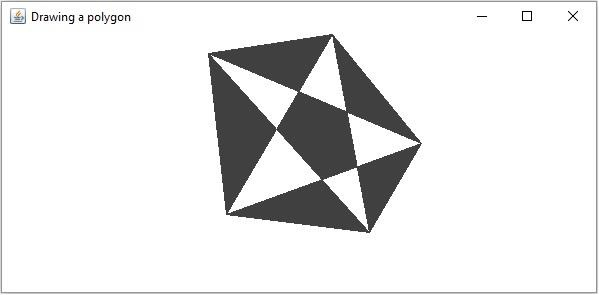# How to draw a filled polygon in OpenCV using Java?

JavaObject Oriented ProgrammingProgramming

#### Complete Java Programming Fundamentals With Sample Projects

98 Lectures 7.5 hours

#### Get your Java dream job! Beginners interview preparation

85 Lectures 6 hours

#### Core Java bootcamp program with Hands on practice

Featured

99 Lectures 17 hours

The org.opencv.imgproc package of Java OpenCV library contains a class named Imgproc. To draw a filled polygon you need to invoke the fillPoly() method of this class. This method accepts the following parameters −

• A Mat object representing the image on which the polygon is to be drawn.

• A-List object holding the objects of the type MatOfPoint.

• A Scalar object representing the color of the polygon.

• An integer representing the line type.

## Example

import java.util.ArrayList;
import java.util.List;
import org.opencv.core.Core;
import org.opencv.core.Mat;
import org.opencv.core.MatOfPoint;
import org.opencv.core.Point;
import org.opencv.core.Scalar;
import org.opencv.highgui.HighGui;
import org.opencv.imgcodecs.Imgcodecs;
import org.opencv.imgproc.Imgproc;
public class DrawingFilledPolygon {
public static void main(String args[]) {
//Reading the source image in to a Mat object
Mat src = Imgcodecs.imread("D:\images\blank.jpg");
//Drawing a polygon
List<MatOfPoint>list = new ArrayList<MatOfPoint>();
new Point(208, 71), new Point(421, 161),
new Point(332, 52), new Point(369, 250),
new Point(421, 161), new Point(226, 232),
new Point(369, 250), new Point(208, 71),
new Point(226, 232), new Point(332, 52)));
Scalar color = new Scalar(64, 64, 64);
int lineType = Imgproc.LINE_8;
Imgproc.fillPoly(src, list, color, lineType);
//Saving and displaying the image
Imgcodecs.imwrite("arrowed_line.jpg", src);
HighGui.imshow("Drawing a polygon", src);
HighGui.waitKey();
}
}

## Output

On executing, the above program generates the following window −